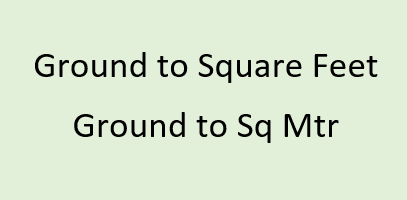# Ground to Square Feet | Ground to Sq Mtr## Ground to Square Feet | Ground to Sq MtrGround is very traditional unit of land area measurement. It was mostly used to measure land area in India. Being a traditional unit of area, its value differs over years. It was earlier approximately equal to 203 square meters (~ 2185 Sq Ft) but commonly it is taken as 2400 Square Feet. Here we will consider all our calculations based on 1 ground = 2400 Square feet. Ground unit is still popular in the context of Real Estate. People generally ask questions like 1 Ground is equal to how many square feet ? or 1 ground is equal to how many square meters ? or convert ground to other units of measurement.
1 Ground = 2400 Square Feet = 222.9673 Square Meters

Convert Ground to other units of Land area measurement :
 Value Convert from Ground Sq. Centimeters Sq. Feet Sq. Yards Hectares Acres Sq. Meters Sq. Kilometers Sq. Miles Cent Convert to Ground Sq. Centimeters Sq. Feet Sq. Yards Hectares Acres Sq. Meters Sq. Kilometers Sq. Miles Cent

Area Conversion of Ground to other Units and vice versa:
1 Ground = 0.0550964187327824 Acre
1 Ground = 0.0222967296 Hectare
1 Ground = 2400 Sq. Feet
1 Ground = 222.967296 Sq. Meter
1 Ground = 0.000222967296 Sq. Kilometer
1 Ground = 266.666666666667 Sq. Yard
1 Ground = 5.50964187327824 Decimal
1 Acre = 18.15 Ground
1 Hectare = 44.8496267362905 Ground
1 Sq. Feet = 0.000416666666666667 Ground
1 Sq. Meter = 0.00448496267362905 Ground
1 Sq. Kilometer = 4484.96267362905 Ground
1 Sq. Yard = 0.00375 Ground
1 Decimal = 0.1815 Ground

Q. 1 Acre consists of how many Ground
A. 1 Acre consists of 18.15 Ground

Q. Convert Ground to Square Yards
A. 1 Ground is equal to 266.6667 Square Yards

Q. 1 Ground is how many sq mtrs?
A. 1 Ground = 222.967296 Sq. Meter

Q. 1 Hectare is how many Ground ?
A. 1 Hectare = 44.8496267362905 Ground